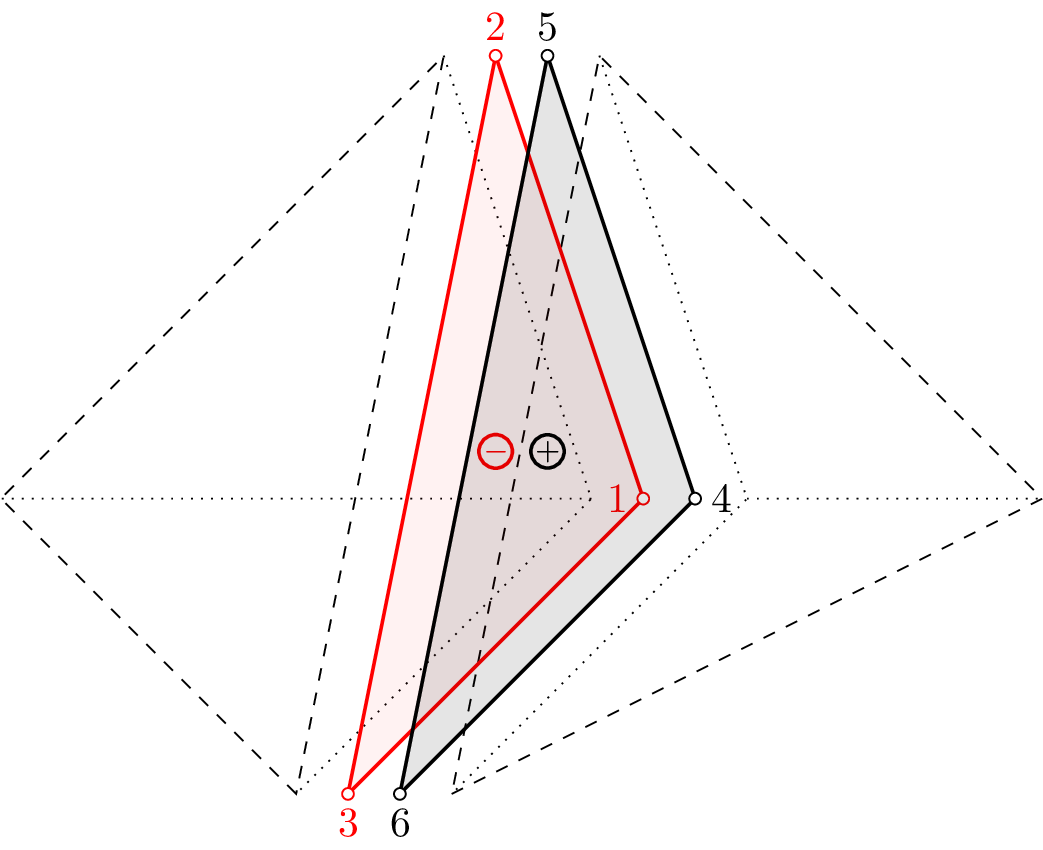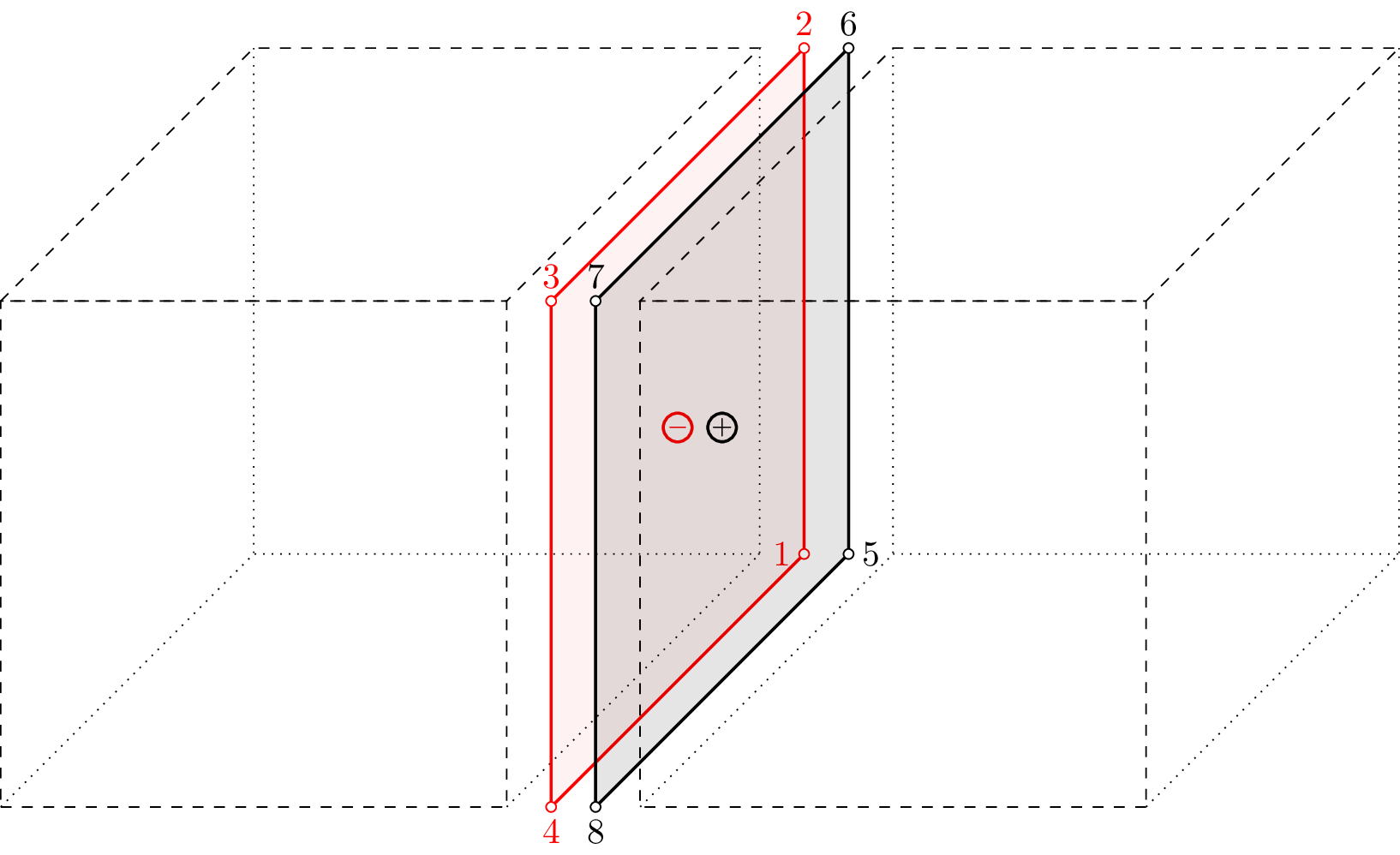#### 3.1 2D Elements

##### 3.1.1 Tr1ht element

Implements the linear triangular finite element for heat transfer problems. Each node has 1 degree of freedom. The cross section thickness property is requested form cross section model. The node numbering is anti-clockwise. The element features are summarized in Table 50.Figure 21: Tr1ht element - node and side numbering.

 Keyword Tr1ht Description triangular finite element with linear approximation for heat transfer problems Specific parameters - Unknowns Single dof (T_f - temperature) is required in each node. Approximation Linear approximation of temperature. Integration Integration using one point gauss integration formula. Loads Body loads are supported. Boundary loads are supported and are computed using numerical integration. The side numbering is following. Each i-th element side begins in i-th element node and ends on next element node (i+1-th node or 1-st node, in the case of side number 3). The local positive edge x-axis coincides with side direction, the positive local edge y-axis is rotated 90 degrees anti-clockwise (see fig. (21)). Features - CS properties - Status

Table 50: Tr1ht element summary

##### 3.1.2 Tr1mt element

Isoparametric triangular finite element with linear approximation of moisture. Other features are the same as for Tr1ht in Section 3.1.1.

##### 3.1.3 Tr1hmt element

Isoparametric triangular finite element with linear approximations of temperature and moisture. Other features are the same as for Tr1ht in Section 3.1.1.

##### 3.1.4 Quad1ht element

Represents isoparametric four-node quadrilateral finite element for heat transfer problems. Each node has 1 degree of freedom. Problem should be defined in x,y plane. The cross section thickness property is requested form cross section model. The nodes should be numbered anti-clockwise (positive rotation around z-axis). The element features are summarized in Table 51.Figure 22: Quad1ht element. Node numbering, Side numbering and definition of local edge c.s.(a).

 Keyword Quad1ht Description Isoparametric four-node quadrilateral linear interpolation element for heat transfer problems Specific parameters [NIP #(in)] Parameters NIP: allows to change the default number of integration point used. Unknowns Single dof (T_f - temperature) is required in each node. Approximation Linear approximation of temperature. Integration Integration using gauss integration formula in 4 (the default), 9, or 16 integration points. The default number of integration point used can be overloaded using NIP parameter. Loads Body loads are supported. Boundary loads are supported and computed using numerical integration. The side numbering is following. Each i-th element side begins in i-th element node and ends on next element node (i+1-th node or 1-st node, in the case of side number 4). The local positive edge x-axis coincides with side direction, the positive local edge y-axis is rotated 90 degrees anti-clockwise (see fig. (22)). Features - CS properties - Status

Table 51: Quad1ht element summary

##### 3.1.5 Quad1mt element

Isoparametric four-node quadrilateral finite element. Other features are the same as for Quad1ht in Section 3.1.4.

##### 3.1.6 Quad1hmt element

Represents isoparametric four-node quadrilateral finite element for heat and mass (one constituent) transfer problems. Two dofs (T_f - temperature and C_1 - concentration) are required in each node. Linear approximation of temperature and mass concentration. Other features are similar to Quad1 element, see section 3.1.4.